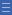# Activity: Happy halving

Activity: Happy halving

Can you split various shapes in half so that the two parts are exactly the same?

## Fing the activity on NRICH

Go to http://nrich.maths.org/217 (link will open in a new window)

## More questions

In how many ways can each shape be halved?

Can you draw a shape that can be halved in exactly 6 ways? Can you draw a non-regular shape that can be halved in exactly 6 ways?

Explain why a regular n-gon can be halved in exactly n ways.

## Shine a light on the Proficiencies

Asking “How many ways?” shows that mathematical problems often have more than answer. An important question to ask is how we know we have found all the possible answers, which highlights the importance of reasoning.

A useful practice to encourage problem solving is to reverse the question. In this case asking students to draw a shape given the number of ways it can be halved encourages problem solving and creative thinking, as well as leading to a possible generalization that regular polygons with n sides can be halved in n ways. The explanation for this requires some careful reasoning as the way in which the polygon is halved depends on whether it has an odd or even number of sides.

Using the term halving provides an introduction to the concept of symmetry.

Yes

Yes

Name Class Section PriorityDownload Happy Halving (blank worksheet) File 1